Courses

# Data Interpretation MCQ 2

## 20 Questions MCQ Test General Aptitude for GATE 2020 | Data Interpretation MCQ 2

Description
This mock test of Data Interpretation MCQ 2 for Quant helps you for every Quant entrance exam. This contains 20 Multiple Choice Questions for Quant Data Interpretation MCQ 2 (mcq) to study with solutions a complete question bank. The solved questions answers in this Data Interpretation MCQ 2 quiz give you a good mix of easy questions and tough questions. Quant students definitely take this Data Interpretation MCQ 2 exercise for a better result in the exam. You can find other Data Interpretation MCQ 2 extra questions, long questions & short questions for Quant on EduRev as well by searching above.
QUESTION: 1

Solution:
QUESTION: 2

Solution:
QUESTION: 3

### The following pie-charts show the distribution of students of graduate and post-graduate levels in seven different institutes in a town. Distribution of students at graduate and post-graduate levels in seven institutes: Total number of students of graduate level = 27300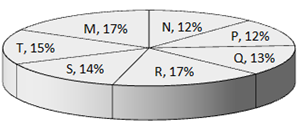Total number of students of post – graduate level = 24700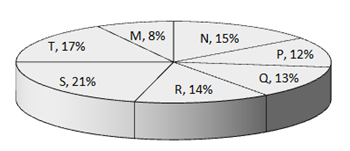Q. How many students of institutes of M and S are studying at graduate level?

Solution:
QUESTION: 4

The following pie-charts show the distribution of students of graduate and post-graduate levels in seven different institutes in a town.
Total number of students of graduate level = 27300Total number of students of post – graduate level = 24700Q.

What is the ratio between the number of students studying at post-graduate level from institutes S and the number of students   studying at graduate level from institute Q?

Solution:
QUESTION: 5

The following pie-charts show the distribution of students of graduate and post-graduate levels in seven different institutes in a town.
Total number of students of graduate level = 27300Total number of students of post – graduate level = 24700Q.

Total number of students studying at post-graduate level from institutes N and P is

Solution:
QUESTION: 6

Study the following line graph and answer the questions.

Exports from Three Companies Over the Years (in Rs. crore)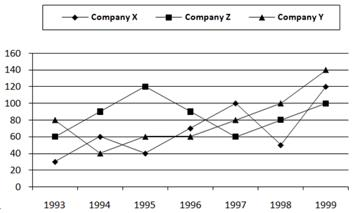Q.

For which of the following pairs of years the total exports from the three Companies together are equal?

Solution:
QUESTION: 7

Study the following line graph and answer the questions.

Exports from Three Companies Over the Years (in Rs. crore)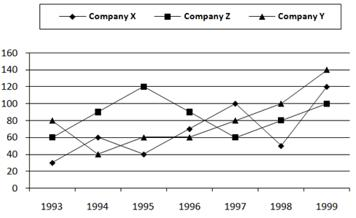Q.
Average annual exports during the given period for Company Y is approximately what percent of the average annual exports for Company Z?

Solution:
QUESTION: 8

Study the following line graph and answer the questions.

Exports from Three Companies Over the Years (in Rs. crore)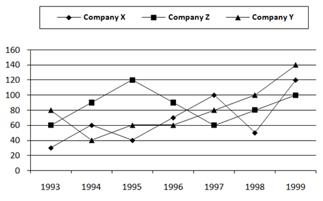Q.
In which year was the difference between the exports from Companies X and Y the minimum?

Solution:
QUESTION: 9

Study the following line graph and answer the questions.

Exports from Three Companies Over the Years (in Rs. crore)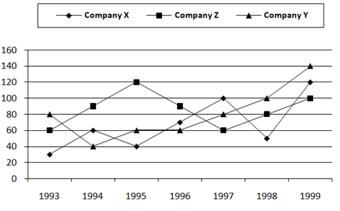Q.
What was the difference between the average exports of the three Companies in 1993 and the average exports in 1998?

Solution:
QUESTION: 10

Study the following line graph and answer the questions.

Exports from Three Companies Over the Years (in Rs. crore)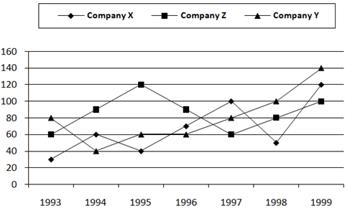Q.
In how many of the given years, were the exports from Company Z more than the average annual exports over the given   years?

Solution:

Average annual exports of Company Z during the given period
= 1/7 x (60 + 90 + 120 + 90 + 60 + 80 + 100)
= Rs. ( 600 /7)crores
= Rs. 85.71 crores.
From the analysis of graph the exports of Company Z are more than the average annual exports of Company Z (i.e., Rs. 85.71 crores) during the years 1994, 1995, 1996 and 1999, i.e., during 4 of the given years.

QUESTION: 11

Study the following line graph and answer the questions based on it.

Number of Vehicles Manufactured by Two companies over the Years (Number in Thousands)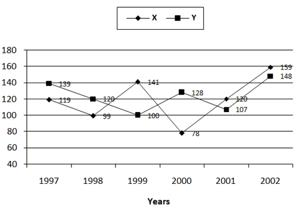Q.
What is the difference between the number of vehicles manufactured by Company Y in 2000 and 2001?

Solution:
QUESTION: 12

Study the following line graph and answer the questions based on it.

Number of Vehicles Manufactured by Two companies over the Years (Number in Thousands)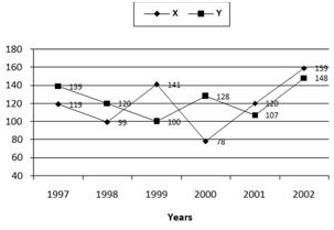Q.
What is the difference between the total productions of the two Companies in the given years?

Solution:
QUESTION: 13

Study the following line graph and answer the questions based on it.

Number of Vehicles Manufactured by Two companies over the Years (Number in Thousands)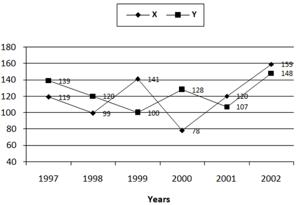Q.
What is the average numbers of vehicles manufactured by Company X over the given period? (rounded off to nearest integer)

Solution: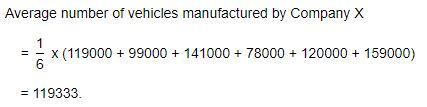QUESTION: 14

Study the following line graph and answer the questions based on it.

Number of Vehicles Manufactured by Two companies over the Years (Number in Thousands)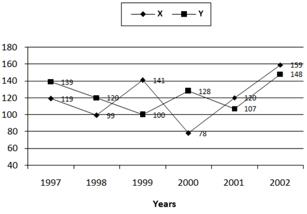Q.
In which of the following years, the difference between the productions of Companies X and Y was the maximum among the   given years?

Solution:
QUESTION: 15

Study the following line graph and answer the questions based on it.

Number of Vehicles Manufactured by Two companies over the Years (Number in Thousands)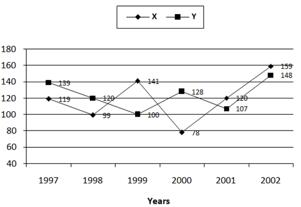Q.
The production of Company Y in 2000 was approximately what percent of the production of Company X in the same year?

Solution:
QUESTION: 16

The following line graph gives the percent profit earned by two Companies X and Y during the period 1996 - 2001.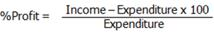Percentage profit earned by Two Companies X and Y over the Given Years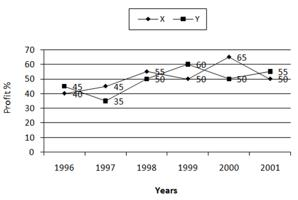Q.
The incomes of two Companies X and Y in 2000 were in the ratio of 3:4 respectively. What was the respective ratio of their   expenditures in 2000?

Solution:
QUESTION: 17

The following line graph gives the percent profit earned by two Companies X and Y during the period 1996 - 2001.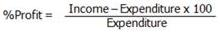Percentage profit earned by Two Companies X and Y over the Given Years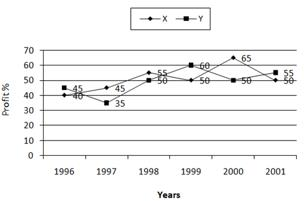Q.
If the expenditure of Company Y in 1997 was Rs. 220 crores, what was its income in 1997?

Solution:
QUESTION: 18

The following line graph gives the percent profit earned by two Companies X and Y during the period 1996 - 2001.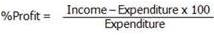Percentage profit earned by Two Companies X and Y over the Given Years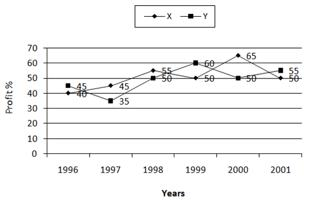Q.
If the expenditures of Company X and Y in 1996 were equal and the total income of the two Companies in 1996 was Rs. 342   crores, what was the total profit of the two Companies together in 1996? (Profit = Income - Expenditure)

Solution:
QUESTION: 19

The following line graph gives the percent profit earned by two Companies X and Y during the period 1996 - 2001.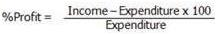Percentage profit earned by Two Companies X and Y over the Given Years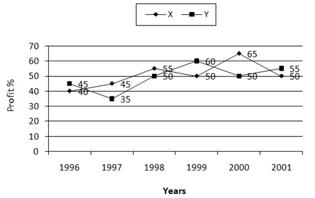Q.
The expenditure of Company X in the year 1998 was Rs. 200 crores and the income of company X in 1998 was the same as its expenditure in 2001. The income of Company X in 2001 was ?

Solution:
QUESTION: 20

The following line graph gives the percent profit earned by two Companies X and Y during the period 1996 - 2001.Percentage profit earned by Two Companies X and Y over the Given Years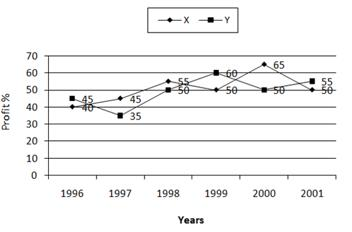Q.
If the incomes of two Companies were equal in 1999, then what was the ratio of expenditure of Company X to that of Company Y in 1999 ?

Solution: